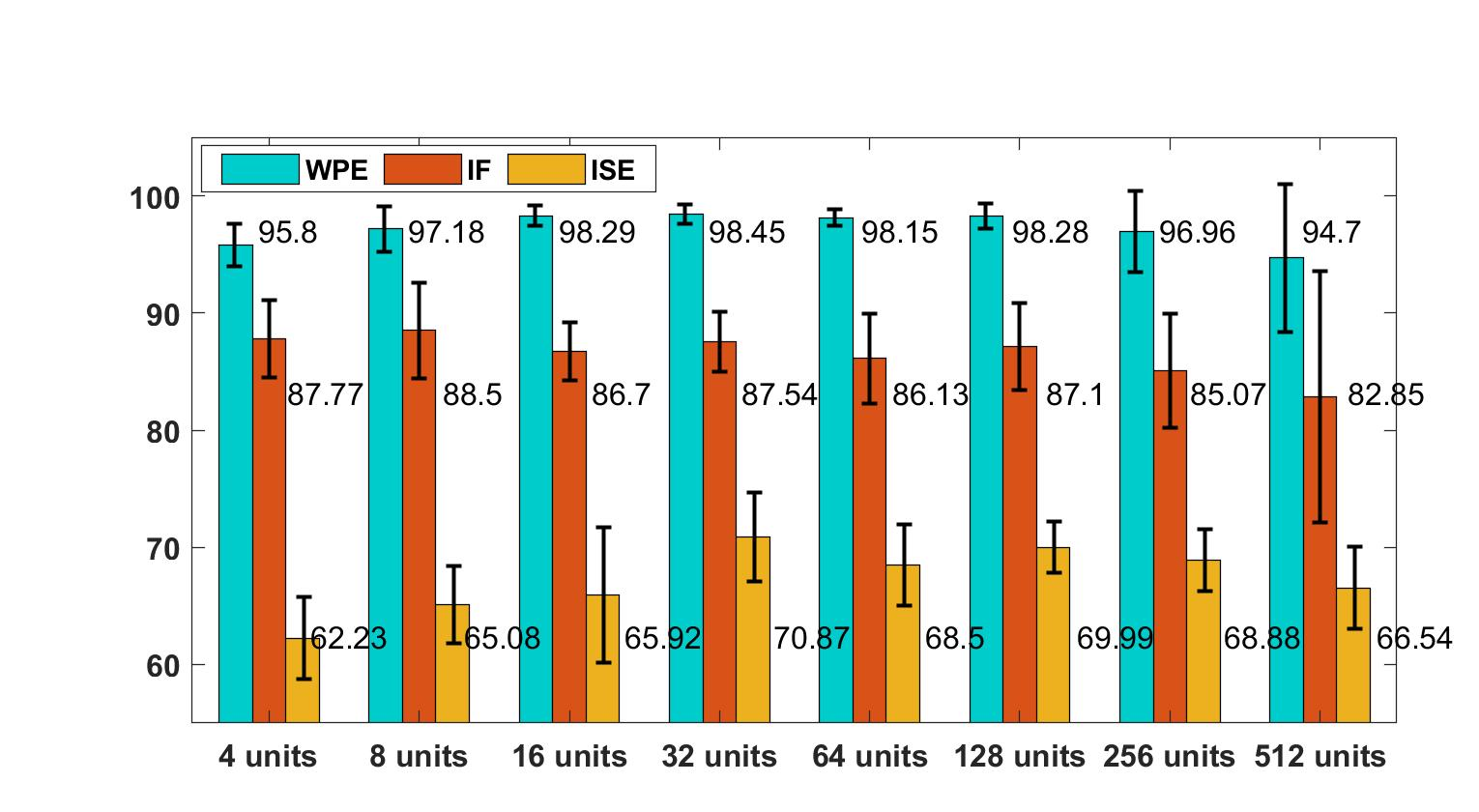• 默认情况下，plotAverage - 计算沿 y 的平均值（对于沿 x 的给定或估计点） - 用平均值的标准误差绘制误差线- 丢弃异常值以计算稳健平均值- 将平均线绘制到数据的轴上（而不是新图） 所有这些选项都可以更改。 ...
• 为了获得最佳分箱：在运行之前将 x 和 y 转换为百分位数亲爱的请参阅 matlab 中心的 boxpdf。 http://www.imt.liu.se/~magnus/cca/tutorial/node16.html Aslak Grinsted 2006 年 2 月http://www.glaciology.net ...
• 利用matlab程序，读取海表面温度卫星影像，并绘制温度分布
• PAGE PAGE 1 matlab中最基本的函数plot的用法 标签matlabplot指令 5.1二维平面图形 5.1.1基本图形函数 plot是绘制二维图形的最基本函数它是针对向量或矩阵的列来绘制曲线的也就是 说使用plot函数之前必须首先定义好...
• 五点滑动平均滤波程序，s正弦波、n噪声、x=s+n，y为五点滑动平均的结果。 ssfile.wav: fs=8000Hz的s正弦波数据，xzfile.wav：fs=8000Hz的...观察数据既可以在matlab中观察，也可以播放出来然后通过虚拟示波器观察数据。
• MATLAB绘制实验数据折现   在论文或者文章写作中，经常需要使用图形来表示我们的实验结果。一般来说，这种表示方式比表格更加直观、更加可视化。因此，本文给出一种使用MATLAB处理数据得到折线的教程。 1. 待...
MATLAB绘制实验数据折现图
在论文或者文章写作中，经常需要使用图形来表示我们的实验结果。一般来说，这种表示方式比表格更加直观、更加可视化。因此，本文给出一种使用MATLAB处理数据得到折线图的教程。
1. 待处理数据形式
待处理的数据为迭代次数与SR、time、RC、length、steerNum、steerAngle、validNode这七个指标的走势图。即随着迭代次数的增加，这七个指标的走势情况。 并且，实验数据包含一个改进和两个对比，三个数据都保存在txt文件中，如下所示。
dealmeans-RRTstar.txt

iteration SR time RC lengthsteerNum steerAngle validNode 150 0 nan nan nan nan nan nan 300 0 nan nan nan nan nan nan 500 0 nan nan nan nan nan nan 1000 0.142857 0.291912 0.0962069 344.143 46.8571 816.639 383.286 1500 0.469388 0.831647 0.107638 347.348 47.6957 923.343 607.478 2000 0.693878 0.726488 0.100066 345.912 46.8235 842.842 753.235 2500 0.816327 0.826433 0.104683 348.025 46.8 883.326 953.75 3000 0.877551 1.11224 0.108346 352.163 47.9535 934.25 1100.91 3500 0.877551 1.61856 0.104273 347.256 47.4884 889.123 1299.21

dealmeans-BRRTstar.txt

iteration SR time RC lengthsteerNum steerAngle validNode 150 0 nan nan nan nan nan nan 300 0 nan nan nan nan nan nan 500 0.0816327 0.108026 0.113985 336.5 48 977.567 229 1000 0.673469 0.306055 0.118107 346.394 49.3636 1049.82 426 1500 1 0.617184 0.112612 343.204 48.8571 991.233 601.694 2000 1 1.2277 0.112168 340.429 47.9184 968.744 773.102 2500 1 1.82997 0.113759 337.306 47.1429 966.036 934.673 3000 1 2.46895 0.111037 334.633 47.2041 944.087 1113.04 3500 1 3.27609 0.112627 333.735 46.9592 951.161 1315.67

dealmeans-BV-RRTstar.txt

iteration SR time RC lengthsteerNum steerAngle validNode 150 1 0.0715696 0.155344 332.959 23.3469 653.243 140.98 300 1 0.181333 0.15514 333.612 25.449 710.931 234.49 500 1 0.377778 0.149981 333.367 26.4694 714.981 344.408 1000 1 1.16517 0.149679 331.408 28.3061 763.375 611.265 1500 1 2.62397 0.143125 328.735 28.9796 746.574 859.041 2000 1 4.57071 0.140159 325.878 29.6327 746.221 1123.14 2500 1 6.14498 0.132265 324.245 30.6531 728.807 1370.67 3000 1 8.19425 0.12972 322.449 30.8776 721.298 1626.59 3500 1 10.9185 0.124152 320.714 30.8163 689.744 1864.41

2. 绘制折线图的MATLAB代码
绘制图形的代码流程为：读取文件，绘制图形，设置xy坐标轴和图例。
close all;
clc;
%只是第一行有字符，则使用importdata可直接读取数据
data1=importdata('D:\大论文试验\实验图片\第四章\补充密集障碍物地图中的实验\dealmeans-RRTstar.txt');
data11=data1.data; %若没有这一步，出不出来图。
data2=importdata('D:\大论文试验\实验图片\第四章\补充密集障碍物地图中的实验\dealmeans-BRRTstar.txt');
data21=data2.data; %若没有这一步，出不出来图。
data4=importdata('D:\大论文试验\实验图片\第四章\补充密集障碍物地图中的实验\dealmeans-BV-RRTstar.txt');
data41=data4.data; %若没有这一步，出不出来图。
x=data11(:,1);
y11=data11(:,2);
y12=data11(:,3);
y13=data11(:,4);
y14=data11(:,5);
y15=data11(:,6);
y16=data11(:,7);
y17=data11(:,8);

y21=data21(:,2);
y22=data21(:,3);
y23=data21(:,4);
y24=data21(:,5);
y25=data21(:,6);
y26=data21(:,7);
y27=data21(:,8);

y41=data41(:,2);
y42=data41(:,3);
y43=data41(:,4);
y44=data41(:,5);
y45=data41(:,6);
y46=data41(:,7);
y47=data41(:,8);
figure(1); %路径长度曲线
%plot(x,y14,'-bo',x,y24,'-rp',x,y44,'-cs',x,y34,'-g*');
plot(x,y14,'-bo',x,y24,'-rp',x,y44,'-g*','LineWidth',1.2); %在figure(1)中绘制三条曲线，设置折现颜色，粗细
title('迭代次数与路径长度');
xlabel('iteration');
ylabel('Path Length');
lgd1=legend('RRT*','Bi-RRT*','BV-RRT*'); %设置图例
%set(lgd1,'Location','BestOutside');
set(lgd1,'FontName','Times New Roman','FontSize',8) %设置图例的字体
lgd1.ItemTokenSize = [20,20];%设置图例尺寸
figure(2); %执行时间，包括runtime
plot(x,y12,'-bo',x,y22,'-rp',x,y42,'-g*','LineWidth',1.2);
title('迭代次数与执行时间');
xlabel('iteration');
ylabel('time(s)');
lgd2=legend('RRT*','Bi-RRT*','BV-RRT*');
%set(lgd2,'Location','BestOutside');
set(lgd2,'FontName','Times New Roman','FontSize',8)
lgd2.ItemTokenSize = [20,20];
figure(3); %成功率SR
plot(x,y11,'-bo',x,y21,'-rp',x,y41,'-g*','LineWidth',1.2);
title('迭代次数与成功率');
xlabel('iteration');
ylabel('SR');
lgd3=legend('RRT*','Bi-RRT*','BV-RRT*');
%set(lgd3,'Location','BestOutside');
set(lgd3,'FontName','Times New Roman','FontSize',8)
lgd3.ItemTokenSize = [20,20];
figure(4); %有效节点数
plot(x,y17,'-bo',x,y27,'-rp',x,y47,'-g*','LineWidth',1.2);
title('迭代次数与有效节点数');
xlabel('iteration');
ylabel('有效节点数');
lgd4=legend('RRT*','Bi-RRT*','BV-RRT*');
set(lgd4,'Location','BestOutside');
set(lgd4,'FontName','Times New Roman','FontSize',8)
lgd4.ItemTokenSize = [20,20];
figure(5); %转弯次数，转弯角度，路径异常值
subplot(2,2,1); %转弯角度
plot(x,y16,'-bo',x,y26,'-rp',x,y46,'-g*','LineWidth',1.2);
title('迭代次数与路径总转向角度');
xlabel('iteration');
ylabel('路径总转向角度');
subplot(2,2,2); %转弯次数
plot(x,y15,'-bo',x,y25,'-rp',x,y45,'-g*','LineWidth',1.2);
title('迭代次数与路径转弯次数');
xlabel('iteration');
ylabel('路径总转弯次数');
subplot(2,2,3); %路径异常值
plot(x,y13,'-bo',x,y23,'-rp',x,y43,'-g*','LineWidth',1.2);
title('迭代次数与路径异常值');
xlabel('iteration');
ylabel('RC');
legend('RRT*','Bi-RRT*','BV-RRT*');

3. 结果图展示4 总结
本文使用MATLAB绘制折线图适合对数据进行可视化处理。使用MATLAB绘制的图片还有一个好处是可以保存为.emf格式，这样插入word中不会失真。
展开全文数据可视化
• 下载MODIS数据，自动绘制MODIS叶绿素，分析叶绿素空间分布
• boxplot3 绘制均值（红色）、标准误差（蓝色、 分位数0.25和0.75）和标准偏差（黑色） 语法：boxplot3(X,Y) 例子： X=1:2； 是{1}=1:100； Y {2} = 101：200； boxplot3(X,Y)
• 采用两种方法绘制固定二元对称信道（BCS）平均互信息曲线，将多条曲线绘制在同一坐标轴上，
• MATLAB 互信息采集曲线数据整理,使用能量分析攻击的任何方法进行攻击之前，我们都需要获取密码设备在运行时 的能量消耗数据。在本文中，我们搭建了一个仿真实验平台来获取 KLEIN 在加密过程 中的能量消耗数据。
• matlab三点跳跃平均代码电阻带校准 权重值 = 通过从 MATLAB 中的数据游标观察手动选择的值范围的平均值 测试程序 一本书的重量~320g test1 - 一次加载一本书 test2 - 一次卸载一本书 test3 - 一次加载和卸载两本书 ...
• TRMM 3B43月平均降水量Matlab读取，此读取也可用于3B42，3B42读取不需要转换降雨量，删去转换部分代码即可。内含注释
• 在不知道原始数据的情况下绘制图形的平均值（参数的平均值）。 中的线条可能具有不同的 x 值。 使用：meanplot(n) 或 meanplot(gcf)，其中 n 是图形编号。
• 带误差棒的柱状，并标注平均值，matlab代码如下，结果如 // code block clear close all load 'plotuse.mat'; avg=[WPE', IF', ISE']; STD=[WPE_std' IF_std' ISE_std']; x=[[1:8]' [1:8]' [1:8]']; h=bar...
画带误差棒的柱状图，并标注平均值，matlab代码如下，结果如图// code block
clear
close all
avg=[WPE', IF', ISE'];
STD=[WPE_std' IF_std' ISE_std'];
x=[[1:8]' [1:8]' [1:8]'];

h=bar(avg);
ylim([55,100])
set(h,'barwidth',1);
h(1).FaceColor='flat';
h(1).CData=repmat([0 0.8 0.8],8,1);

hold on;
ylim([55,105]);
errorbar(x(:,1)-0.23,avg(:,1),STD(:,1),'linestyle','none','linewidth',1,'color','k');
errorbar(x(:,2),avg(:,2),STD(:,2),'linestyle','none','linewidth',1,'color','k');
errorbar(x(:,3)+0.23,avg(:,3),STD(:,3),'linestyle','none','linewidth',1,'color','k');

str_WPE=num2str(avg(:,1));
str_IF=num2str(avg(:,2));
str_ISE=num2str(avg(:,3));

dim=0.16:0.09:(0.16+7*0.09);
dim_multi_WPE=[dim' repmat([0.65,0.1,0.1],8,1)];
dim=0.18:0.09:(0.18+7*0.09);
dim_multi_IF=[dim', repmat([0.45,0.1,0.1],8,1)];
dim=0.2:0.09:(0.2+7*0.09);
dim_multi_ISE=[dim', repmat([0.15,0.1,0.1],8,1)];
for i=1:8
annotation('textbox',dim_multi_WPE(i,:),'LineStyle', 'none', 'LineWidth',1,'String',str_WPE(i,:),'fontsize',12);
annotation('textbox',dim_multi_IF(i,:),'LineStyle','none','LineWidth',1,'String',str_IF(i,:),'fontsize',12);
a3=annotation('textbox',dim_multi_ISE(i,:),'LineStyle','none','LineWidth',1,'String',str_ISE(i,:));
a3.FontSize=12;
end

set(gca,'xticklabel',{'4 units','8 units','16 units','32 units','64 units','128 units','256 units','512 units'},'fontsize',12,'fontweight','bold');
legend([h(1),h(2),h(3)],{'WPE', 'IF','ISE'},'location','northwest','NumColumns',3);

展开全文• MATLAB 练习计算并显示来自指定语音文件的一帧语音的 AMDF，并基于逐帧使用 AMDF 实现基音检测算法。 该程序可以在帧模式下... 该练习绘制了基音周期轮廓以及基于每个分析帧获得的 AMDF 最小值的强度的置信度量
• 此示例从 Berkeley Earth 加载 World 数据集的 Monthly Climatic Data，并绘制在可用数据集上计算的一年中 12 个月的平均温度。
• 此函数使用半透明背景绘制一组数据的平均值和标准偏差，填充正负平均误差之间的空间，完全可定制。 输入参数： -数据：数据矩阵，其中的行对应于观测值，而列对应于样本。 - 选项：（可选）包含自定义参数的结构。 *...
• function MIhat = nmi( A, B )
• yidongpingjunfa.m采用了背景差法检测运动目标，移动平均法提取图像背景。 minboundrect.m用于绘制运动目标的最小外接矩形框，可任意设置最小外接矩形框的角度。 本人做的是视频中运动车辆的检测，读者可自行更换...
• matlab开发-移动窗口的平均值和方差。移动窗均值和移动窗方差的有效计算
• 径向平均功率谱（RAPS）是与方向无关的平均谱，即所有... 此函数计算并绘制输入矩阵的 RAPS（不考虑平均半径外的角值）。 图像可以是矩形的，但必须是二维的（例如，不支持多色通道数据）。 还指定了数据的空间分辨率。
• 基于Matlab曲线拟合的山东省各地市人口密度预测及Cartogram地图表现.pdf
• 小型红外目标检测的绝对方向平均差（ADMD）算法 以下论文的MATLAB和OpenCV两种实现：使用绝对方向均值差算法的快速而强大的小型红外目标检测 如果您在研究中使用这些代码，请引用以下论文： 的MATLAB 您可以在MATLAB...
下载的网址 http://www.hycom.org/dataserver/gofs-3pt1/analysis
%%    画盐度分布图
clear,clc
%读取经纬度
[Lat,Lon]=meshgrid(lat,lon);
%读取盐度
salinity=cat(4,s1,s2);
%去除深度，按时间维度对非nan数做平均
S = nanmean(squeeze(salinity(:,:,1,:)),3);
%画盐度分布图
figure(1)
m_proj('Mercator','lon',[119 129],'lat',[23 33]);
m_contourf(Lon,Lat,S,[25:0.25:32.6]);%画盐度等值线
colormap(jet);colorbar('fontsize',10);
m_gshhs_i('color','k','linewidth',0.2,'linestyle','none');
m_gshhs_i('patch',[.5 .8 0],'edgecolor','none');
m_grid('box','none','xtick',6,'ytick',6,'frontsize',15);
m_text(127,24,'salinity','color','w','fontsize',15);
title('6月份东海盐度分布图')

%% 画流场图
[Lat,Lon]=meshgrid(lat,lon);
%读取u,v数据

U = nanmean(squeeze(u(:,:,1,:)),3);
V = nanmean(squeeze(v(:,:,1,:)),3);
speed = sqrt(U.^2+V.^2);
Speed=speed(:);Lon=Lon(:);Lat=Lat(:);U=U(:);V=V(:);

ind=find(Speed==0);
Speed(ind)=nan;Lon(ind)=nan;Lat(ind)=nan;U(ind)=nan;V(ind)=nan;

Speed=ceil(Speed*100);
%保留U,V,Lon,Lat,Speed与(Speed中的非nan值相应位置)的数值
U=U(~isnan(Speed));V=V(~isnan(Speed));
Lon=Lon(~isnan(Speed));Lat=Lat(~isnan(Speed));
Speed=Speed(~isnan(Speed));
mm=flipud(hot(130));

figure(2)
m_proj('Mercator','lon',[119 129],'lat',[23 33]);
%画地形水深图
[CS,CH]=m_etopo2('contourf',[-7000:500:3000],'edgecolor','none');
m_gshhs_i('color','k','linewidth',0.2,'linestyle','none');
m_gshhs_i('patch',[.5 .8 0],'edgecolor','none');
m_grid('box','none','xtick',6,'ytick',6,'frontsize',15);
hold on
%画流速矢量图
for j=1:5:length(Speed)
if Speed(j)<=130
m_quiver(Lon(j),Lat(j),U(j),V(j),0.5,'color',mm(Speed(j),:),...
else
m_quiver(Lon(j),Lat(j),U(j),V(j),0.5,'color',mm(130,:),...
end
end
title('6月份东海流场分布图')

展开全文• matlab开发-用高亮度分位数绘制数据的正常分布绘制数据的直方，使其与突出显示分位数的正态分布相匹配
• 包中提供的示例文件 (global_mean_sea_level_plotter.m) 使用 get_gmsl 和 get_gmsl_ns 绘制从 1992 年到呈现的全球平均海平面，并计算拟合数据的线性最小二乘法。 数据来自科罗拉多大学海平面研究小组网站， ...
• MATLAB 科研绘图--创建柱状
创建柱状图
对于绝大多数的情况，统计对象是一组离散的数据，我们要计算他们的平均值，方差，标准差等。数据可以用柱状图呈现出来，现在我们来看一个例子： 假设某班有36位同学，学生的期末考试成绩为： 100分–1人 96分–2人 90分–4人 88分–2人 85分–3人 84分–1人 82分–2人 78分–7人 75分–4人 70分–6人 69分–1人 63分–2人 55分–1人 我们在MATLAB所做的第1件事就是输入数据，输入分数x和得到该分数的人数y:
>> x = [55 63 69 70 75 78 82 84 85 88 90 96 100];
>> y = [1 2 1 6 4 7 2 1 3 2 4 2 1];

然后生成柱状图：但这远远不够，我们看到x轴许多数据重合，我们想看到某个分数区间的人数，比如50~59分之间，我们还要对我们的图像进行修改。 现在我们要对成绩区间进行划分： 一位学生在50～59分之间 三位学生在60～69分之间 17位学生在70～79分之间 8位学生在80～89分之间 7位学生在90～100分之间 接下来我们创建两个数组，一个用来存储每个分数段的中间值，另一个存放每个分数段的人数。
>> x = [54.5 64.5 74.5 84.5 94.5];
>> y = [1 3 17 8 7];
>> bar(x,y),xlabel('分数'),ylabel('学生人数'),title('期末考试成绩')

更加专业;其它的命令：
barh:产生水平的柱状图bar3:三维图像bar3h:三维水平图像例子： 有三个班，分别由三个老师教学，其中在不同的分数段有不同的人数，创建出带有多种数据集合的柱状图可以组合和堆和：  bar(x,y,‘grouped’)  由于grouped是默认选项，所以我们还可以写成bar(x,y)
  bar(x,y,‘stacked’)
我们输入的数据是一个多列数组，第一列代表A班成绩分布，以此列类推：
>> x = [54.5 64.5 74.5 84.5 94.5];
>> A = [0;3;18;13;10];...
B = [3;5;20;10;5];...
C = [1;2;15;17;8];
>> y = [A B C];
>> bar(x,y),xlabel('考试分数'),ylabel('学生人数'),legend('A','B','C');展开全文java 数据分析 大数据 python
• 平均梯度就是一幅图像的梯度上所有点的均值。它反映了图像中的微小细节反差和纹理变化特征，同时也能反映出图像的清晰度。一般来说平均梯度越大，图像层次也就越丰富，变化就越多，图像也就越清晰。所以我们可以把...
• Matlab 绘图（一）圆形绘制 最近在用MATLAB做一些机构的运动可视化，用到了一些动画和绘图的东西，在网上查了一些资料，特意在这里记录一下。首先是MATLAB中圆形的绘制，常见的有三种方式： rectangle函数 ...可视化
• 一、源代码 >> a=[8 9 10 7 8 9];%mean >> b=[1 2 1 3 1 1]%std b =  1 2 1 3 1 1 >> figure(); >> bar(a,'c'); >> hold on; >> errorbar(a,b,'k','LineStyle','none'); ...二、效果...

# matlab画平均图matlab 订阅